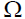1. Consider the circuit shown below. In a certain steady state, Y is at logical ‘l’. What are possible values of A, B, C ?(A) A = 0, B = 0, C = 1
(B) A = 0, B = C = 1
(C) A = 1, B = C = 0
(D) A = B = 1, C = 1

2. The worst case time complexity of AVL tree is better in comparison to binary search tree for
(A) Search and Insert Operations
(B) Search and Delete Operations
(C) Insert and Delete Operations
(D) Search, Insert and Delete Operations

3. The GSM network is divided into the following three major systems :
(A) SS, BSS, OSS

(B) BSS, BSC, MSC
(C) CELL, BSC, OSS
(D) SS, CELL, MSC

4. The power set of the set {} is
(A) {}
(B) {, {}}
(C) {0}
(D) {0,, {}}

5. If the disk head is located initially at 32, find the number of disk moves required with FCFS if the disk queue of I/O blocks requests are 98, 37, 14, 124, 65, 67.
(A) 239
(B) 310
(C) 321
(D) 325

6. Component level design is concerned with
(A) Flow oriented analysis
(B) Class based analysis
(C) Both of the above
(D) None of the above

7. The ‘C’ language is
(A) Context free language
(B) Context sensitive language
(C) Regular language
(D) None of the above

8. The Mobile Application Protocol (MAP) typically runs on top of which protocol ?
(A) SNMP (Simple Network Management Protocol)
(B) SMTP (Simple Mail Transfer Protocol)
(C) SS7 (Signalling System 7)
(D) HTTP (Hyper Text Transfer Protocol)

9. If a packet arrive with an M-bit value is ‘l’ and a fragmentation offset value ‘0’, then it is ______ fragment.
(A) First

(B) Middle
(C) Last
(D) All of the above

10. The number of bit strings of length eight that will either start with a 1 bit or end with two bits 00 shall be
(A) 32
(B) 64
(C) 128
(D) 160

11. In compiler design ‘reducing the strength’ refers to
(A) reducing the range of values of input variables.
(B) code optimization using cheaper machine instructions.
(C) reducing efficiency of program.
(D) None of the above

12. In which addressing mode, the effective address of the operand is generated by adding a constant value to the contents of register ?
(A) Absolute
(B) Indirect
(C) Immediate
(D) Index

13. Which of the following is true ?

(A) A relation in BCNF is always in 3NF.
(B) A relation in 3NF is always in BCNF.
(C) BCNF and 3NF are same.
(D) A relation in BCNF is not in 3NF.

14. Given memory partitions of 100 K, 500 K, 200 K, 300 K and 600 K (in order) and processes of 212 K, 417 K, 112 K, and 426 K (in   order), using the first-fit algorithm, in which partition would the process requiring 426 K be placed ?
(A) 500 K
(B) 200 K
(C) 300 K
(D) 600 K

15. What is the size of the Unicode character in Windows Operating System ?
(A) 8-Bits
(B) 16-Bits
(C) 32-Bits
(D) 64-Bits

16. In which tree, for every node the height of its left subtree and right subtree differ almost by one ?
(A) Binary search tree
(B) AVL tree
(D) Complete Binary Tree

17. The design issue of Datalink Layer in OSI Reference Model is
(A) Framing
(B) Representation of bits
(C) Synchronization of bits
(D) Connection control

18. Given the following expressions of a grammar
E -> E * F / F + E / F
F -> F – F / id
Which of the following is true ?

(A) * has higher precedence than +
(B) – has higher precedence than *
(C) + and – have same precedence
(D) + has higher precedence than *

19. The maturity levels used to measure a process are
(A) Initial, Repeatable, Defined, Managed, Optimized.
(B) Primary, Secondary, Defined, Managed, Optimized.
(C) Initial, Stating, Defined, Managed, Optimized.
(D) None of the above

20. The problem of indefinite blockage of low-priority jobs in general priority scheduling algorithm can be solved using :
(A) Parity bit
(B) Aging
(C) Compaction
(D) Timer

21. Which API is used to draw a circle ?
(A) Circle ( )
(B) Ellipse ( )
(C) Round Rect ( )
(D) Pie ( )

22. In DML, RECONNCT command cannot be used with
(A) OPTIONAL Set
(B) FIXED Set
(C) MANDATOR Set
(D) All of the above

23. Coaxial cables are categorized by Radio Government rating are adapted for specialized functions. Category RG-59 with impedance 75used for
(A) Cable TV

(B) Ethernet
(C) Thin Ethernet
(D) Thick Ethernet